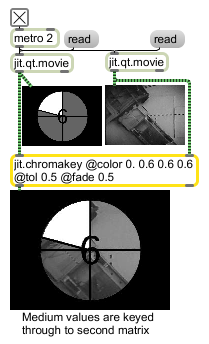jit.chromakey

Keying based on chromatic distance

Description

The jit.chromakey object measures the chromatic distance of each of the left input's cells (pixels) with a reference color (a.k.a "green screening"). The total chromatic distance is calculated by summing the absolute value of each color channel's distance from the reference color's corresponding color channel.

If the distance is less than or equal to a tolerated distance ( tol ) value, the right input cell is multiplied by a maximum keying ( maxkey ) value.

If the distance is greater than the tolerated distance but less than the sum of the tolerated value and a set fade value ( fade ) an interpolated value between the some value between minkey and maxkey values is applied.

For values whose distance is greater than the sum of the tolerated and fade values, the right input cell is multiplied by the minkey value.

The left input is always multiplied by the additive inverse of the left input's multiplicand.

The jit.chromakey object has two modes of output; the first keys between the two input sources, and the second mode will output the keying values (i.e., the left input multiplicands) rather than applying the keying -- the left input is ignored. This is useful for outputting keying information for use with other Jitter objects. Keying can be inverted by swapping the minkey and maxkey values.

Matrix Operator

matrix inputs:2, matrix outputs:1
 Name IOProc Planelink Typelink Dimlink Plane Dim Type in2 resamp 1 1 1 1 1 char long float32 float64 out n/a 1 1 1 1 1 char long float32 float64

Attributes

Name Type g/s Description
alphaignore int Ignore alpha channel flag (default = 1)
0 = use alpha channel (plane 0) when calculating chromatic distance
0 = ignore alpha channel (plane 0) when calculating chromatic distance
color float The reference color to be used for keying (default = all 0.)
maxkey float The maximum keying value (default = 1.)
minkey float The minimum keying value (default = 0.)
mode int Output mode (default = 0)
0 = perform keying and output matrix
1 = output the key map for use elsewhere
2 = alpha contains key map, other planes are from left input
tol float The tolerated distance -- the range of variance from which an input cell's absolute value can diverge when calculating keying (default = 0.)

Examples